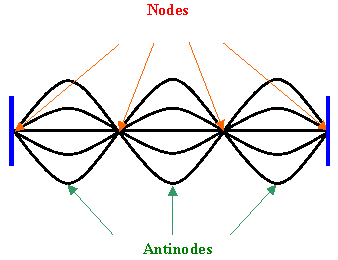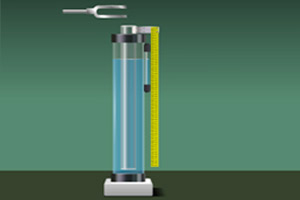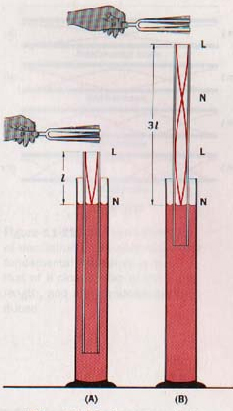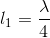Resonance Column

# Objective

To find the velocity of sound in air at room temperature using the resonance column by determining two resonance positions. Also find the velocity of sound in air at 00C.

# Theory

## Stationary wavesStationary waves are produced by the superposition of two waves of same frequency and amplitude travelling with same velocity in opposite directions. Due to the constructive interference, these waves produce certain fixed points along the medium which undergo zero displacement. These points of no displacement are known as nodes. Midway between every nodes are regions of maximum displacement. These points are called antinodes.

## Production of longitudinal stationary waves in air columnStanding waves can be formed in a tube of air due to the interference of longitudinal sound waves travelling in opposite directions. In a pipe closed at one end, the closed end is a displacement node and the open end is a displacement antinode.

Vibration of air column can be set up in a resonance column apparatus. It consists of a long metal tube held vertically in a tall jar containing the water. The tube can be fixed in vertical position. The length of the air column can be varied by raising or lowering the tube.Here, the surface of water will act as the closed end. When a vibrated tuning fork is held above the open end, longitudinal waves are sent down the air column. These waves are reflected at the water surface and thus produce standing waves. Nodes are produced at the water surface and antinodes are produced at the open end.

When the frequency of waves in the air column becomes equal to the natural frequency of tuning fork, a loud sound is produced in the air column. It is the condition for resonance. It occurs only when the length of air column is proportional to one-fourth of the wavelength of sound waves having frequency equal to frequency of tuning fork.

ie;   For first resonance,For second resonance,From the above equations we get the relation,Thus the velocity of sound waves on air at room temperature can be found as,ie;which can be calculated.

If v be the velocity of sound in air at temperature t°C, then the velocity of sound at 0°C can be calculated as,# Learning Outcomes

• Students understand the concept of standing waves and the various terms related to them.
• Students learn the relation between frequency, wavelength and velocity of a wave.
• Students understand transverse and longitudinal waves.

Cite this Simulator: